# Dispersion Compensation with Optical Phase Conjugation (OPC)

Although the use of optical phase conjugation (OPC) for dispersion compensation was proposed in 1979, it was only in 1993 that the OPC technique was first implemented experimentally. It has attracted considerable attention since then. In contrast to other optical schemes discussed in previous tutorials, the OPC is a nonlinear optical technique. This tutorial describes the principle behind it and discusses its implementation in practical lightwave systems.

#### 1. Principle of Operation

The basic idea behind any dispersion-management scheme is quite simple and can be understood from the pulse-propagation equation used in this dispersion-induced pulse broadening tutorial for studying the impact of fiber dispersion and written here aswhere β3 governs the effects of third-order dispersion (TOD).

The simplest way to understand how OPC can compensate the GVD is to take the complex conjugate of the above equation and obtain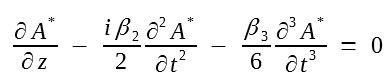A comparison of the two equations above shows that the phase-conjugated field A* propagates with the sign reversed for the GVD parameter β2. This observation suggests immediately that, if the optical field is phase-conjugated in the middle of the fiber link, as shown in figure (a) below, second-order dispersion (GVD) accumulated over the first half will be compensated exactly in the second half of the fiber link. As the β3 term does not change sign on phase conjugation, OPC cannot compensate for the third-order dispersion (TOD). In fact, it is easy to show by keeping the higher-order terms in the Taylor expansion that OPC compensates for all even-order dispersion terms, while leaving the odd-order terms unaffected.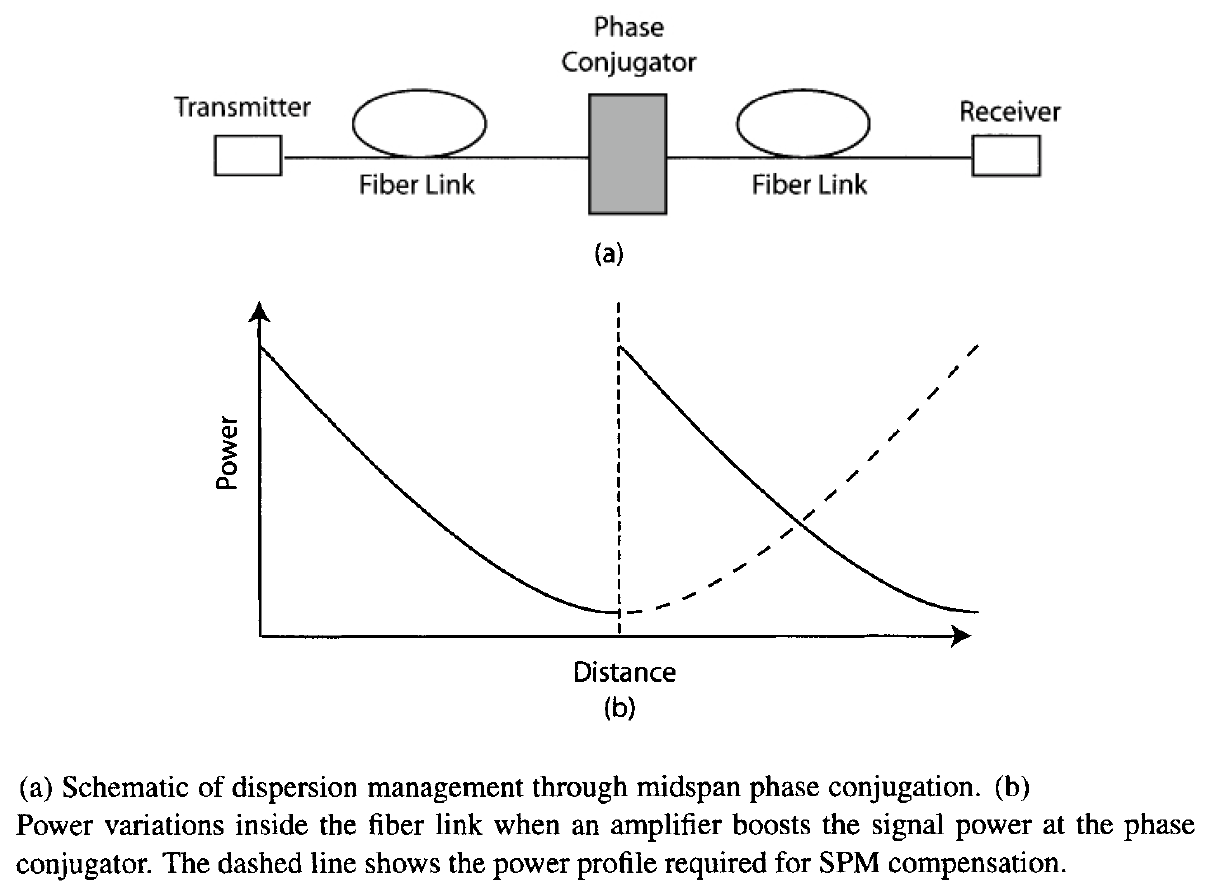The effectiveness of midspan OPC for dispersion compensation can also be verified by using the following equation with β3 = 0 (this equation is the solution to the pulse-propagation equation above).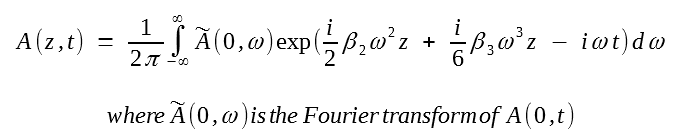The optical field just before OPC is obtained by substituting z = L/2 in this equation. The propagation of the phase-conjugated field A* in the second-half section then yields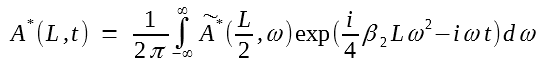where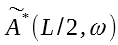is the Fourier transform of A*(L/2, t) and is given by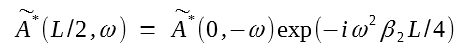By substituting this expression back into the above equation, one finds that A(L, t) = A*(0, t). Thus, except for a phase reversal induced by the OPC, the input field is completely recovered, and the pulse shape is restored to its input form. Since the signal spectrum after OPC becomes the mirror image of the input stream, the OPC technique is also referred to as midspan spectral inversion.

#### 2. Compensation of Self-Phase Modulation

As discussed in the nonlinear optical effects tutorial, the nonlinear phenomenon of self-phase modulation (SPM) leads to the chirping of the transmitted signal that manifests itself through broadening of the signal spectrum. In most lightwave systems, SPM effects degrade the signal quality, especially when the signal is propagated over long distances using multiple optical amplifiers. If turns out that the OPC technique can compensate for both the GVD and SPM simultaneously. This feature of OPC was noted in the early 1980s and attracted considerable attention after 1993.

It is easy to show that both the GVD and SPM are compensated perfectly in the absence of fiber losses. Pulse propagation in a lossy fiber is governed bywhere α accounts for fiber losses. When α = 0, A* satisfies the same equation when we take the complex conjugate of the above equation and change z to -z. In other words, the propagation of A* is equivalent to sending the signal backward and undoing distortions induced by β2 and γ. As a result, midspan OPC can compensate for both SPM and GVD simultaneously.

Fiber losses destroy this important property of midspan OPC. The reason is intuitively obvious if we note that the SPM-induced phase shift is power-dependent. As a result, much larger phase shifts are induced in the first half of the link than the second half, and OPC cannot compensate for the nonlinear effects. But the equation above can be used to study the impact of fiber losses. By making the substitution A(z, t) = B(z, t)p(z), the above equation can be written aswhere p(z) = exp(- αz). The effect of fiber losses is mathematically equivalent to the loss-free case but with a z-dependent nonlinear parameter. By taking the complex conjugate of this equation and changing z to -z, it is easy to see that perfect SPM compensation can occur only if p(z) = exp(αz) after phase conjugation (z > L/2). A general requirement for the OPC technique to work is p(z) = p(L - z). This condition cannot be satisfied when α ≠ 0.

One may think that the problem can be solved by amplifying the signal after OPC so that the signal power becomes equal to the input power before it is launched in the second-half section of the fiber link. Although such an approach reduces the impact of SPM, it does not lead to perfect compensation of it. The reason can be understood by noting that the propagation of a phase-conjugated signal is equivalent to propagating a time-reversed signal. Thus, perfect SPM compensation can occur only if the power variations are symmetric around the midspan point where the OPC is performed so that p(z) = p(L - z) in the equation above. Optical amplification does not satisfy this property. Figure (b) above shows the actual and required form of p(z). One can come close to SPM compensation if the signal is amplified often enough that the power does not vary by a large amount during each amplification stage. This approach is, however, not practical because it requires closely spaced amplifiers. The use of distributed Raman amplification with bidirectional pumping can also help because it can provide p(z) close to 1 over the entire span.

Perfect compensation of both GVD and SPM can be realized by employing dispersion-decreasing fibers in which |β2| decreases along the fiber length. To see how such a scheme can be implemented, assume that β2 in the above equation is a function of z. By making the transformation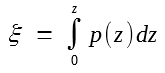the last equation can be written aswhere b(z) = β2(z)/p(z). Both GVD and SPM can be compensated if b(ξ) = b(ξL - ξ), where ξL is the value of ξ at z = L. This condition is automatically satisfied when β2(z) decreases in exactly the same way as p(z) so that their ratio remains constant. Since p(z) decreases exponentially, both GVD and SPM can be compensated in a dispersion-decreasing fiber whose GVD decreases as e-αz. This approach is quite general and applies even when in-line amplifiers are used.

#### 3. Generation of Phase-Conjugated Signal

The implementation of the midspan OPC technique requires a nonlinear optical element that generates the phase-conjugated signal. The most commonly used method makes use of four-wave mixing (FWM) in a nonlinear medium. Since the optical fiber itself is a nonlinear medium, a simple approach is to use a special fiber designed to maximize the FWM efficiency. The use of FWM requires launching of a pump beam at a frequency ωp that is shifted from the signal frequency ωs by a small amount (~0.5 THz). Such a device acts as a parametric amplifier and amplifies the signal, while also generating an idler at the frequency ωc = 2ωp - ωs if the phase-matching condition is satisfied. The idler carries the same information as the signal but is phase is reversed with respect to the signal and its spectrum is inverted.

The phase-matching condition can be approximately satisfied if the zero-dispersion wavelength of the OPC fiber is chosen to nearly coincide with the pump wavelength. This was the approach adopted in a 1993 experiment in which a 1,546-nm signal was phase-conjugated using FWM in a 23-km-long fiber pumped at 1,549 nm. A 6-Gb/s signal could be transmitted over 152 km by compensating dispersion through OPC in the middle of the fiber link. In another 1993 experiment, a 10-Gb/s signal was transmitted over 360 km with the setup shown in the figure below. The midspan OPC was performed in a 21-km-long fiber by using a pump laser whose wavelength was tuned exactly to the zero-dispersion wavelength of the fiber. The pump and signal wavelengths differed by 3.8 nm, and a bandpass filter was used to separate the phase-conjugated signal from the pump.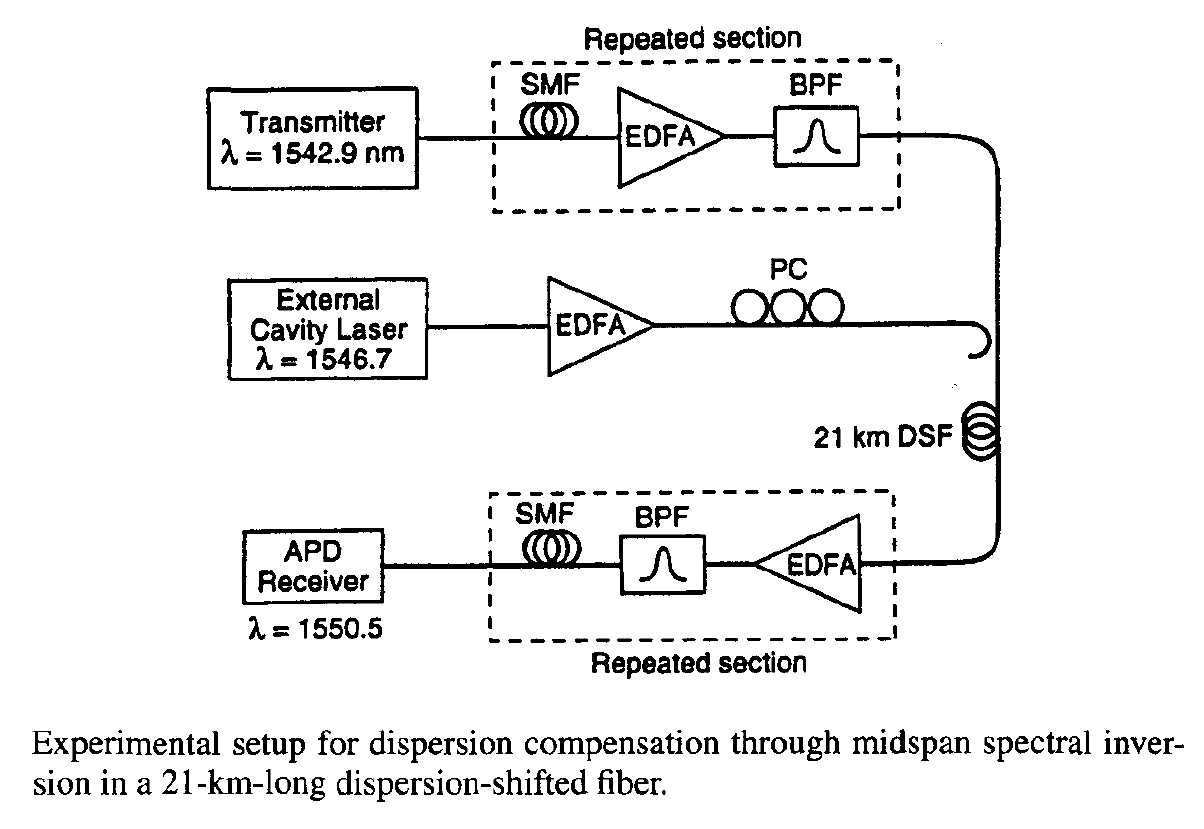Several factors need to be considered while implementing the midspan OPC technique in practice.

First, since the signal wavelength changes from ωs to ωc = 2ωp - ωs at the phase conjugator, the GVD parameter β2 becomes different in the second-half section. As a result, perfect compensation occurs only if the phase conjugator is slightly offset from the midpoint of the fiber link. The exact location Lp can be determined by using the condition β2s)Lp = β2c)(L - Lp), where L is the total link length. By expanding β2c) in a Taylor series around the signal frequency ωs, Lp is found to bewhere δc = ωc - ωs is the frequency shift of the signal induced by the OPC technique. For a typical wavelength shift of 6 nm, the phase-conjugator location changes by about 1%. The effect of residual dispersion and SPM in the phase-conjugator fiber itself can also affect the placement of a phase conjugator.

A second factor that needs to be addressed is that the FWM process in optical fibers is polarization-sensitive. As signal polarization is not controlled in optical fibers, it varies at the OPC in a random fashion. Such random variations affect FWM efficiency and make the standard FWM technique unsuitable for practical purposes. Fortunately, the FWM scheme can be modified to make it polarization-insensitive. In one approach, two orthogonally polarized pump beams at different wavelengths, located symmetrically on the opposite sides of the zero-dispersion wavelength λZD of the fiber, are used. This scheme has another advantage: The phase-conjugate wave can be generated at the frequency of the signal itself by choosing λZD such that it coincides with the signal frequency. Polarization-insensitive OPC can also be realized by using a single pump in combination with a fiber grating and an orthoconjugate mirror, but the device works in the reflective mode and requires separation of the conjugate wave from the signal through an optical circulator.

Low efficiency of the OPC process can be of a concern. In early experiments, the conversion efficiency ηc was below 1% making it necessary to amplify the phase-conjugated signal. However, the FWM process is not inherently a low-efficiency process and, in principle, it can even provide net gain. Indeed, analysis of the FMW equations shows that ηc can be increased considerably by increasing the pump power; it can even exceed 100% by optimizing the power levels and the wavelength difference of the pump and signal. High pump powers require suppression of stimulated Brillouin scattering through modulation of pump phases. In a 1994 experiment, 35% conversion efficiency was realized with this technique.

The FWM process in a semiconductor optical amplifier (SOA) can also be used to generate the phase-conjugated signal. This approach was first used in a 1993 experiment to demonstrate the transmission of a 2.5-Gb/s signal over 100 km of standard fiber. Later, in a 1995 experiment the same approach ws used for transmitting a 40-Gb/s signal over 200 km of standard fiber. The possibility of highly nondegenerate FWM inside SOAs was suggested in 1987, and this technique is used extensively in the context of wavelength conversion. Its main advantage is that the phase-conjugated signal can be generated in a device of 1-mm length. The conversion efficiency is also typically higher than that of FWM in an optical fiber because of amplification, although this advantage is offset by the relatively large coupling losses resulting from the need to couple the signal back into the fiber. By a proper choice of the pump-signal detuning, conversion efficiencies of more than 100% (net gain for the phase-conjugated signal) have been realized through FWM in SOAs.

Periodically poled lithium-niobate (PPLN) waveguides have been used to make a compact, wideband, spectral inverter. The phase-conjugated signal in such a device is generated through two cascaded second-order nonlinear processes that are quasi-phase-matched through periodic poling of the crystal. Such an OPC device exhibited only 7-dB insertion losses and was capable of compensating the dispersion of four 10-Gb/s channels simultaneously over 150 km of standard fiber. By 2003, a PPLN waveguide was used for simultaneous phase conjugation of 103 channels from the C-band to the L-band with a conversion efficiency of about -15 dB using a single pump at 1555 nm.

The OPC technique has been used for dispersion compensation in several recent experiments. In a 2004 WDM experiment, a PPLN-based phase conjugator was used to demonstrate the transmission of 16 channels (each operating at 40 Gb/s) over 800 km of standard fiber. Amplifier spacing was 100 km and a single PPLN device was used midway after 4 amplifiers. The figure below shows the experiment setup schematically. A single pump at a wavelength of 1,546.12 nm phase-conjugated all 16 WDM channels at it inverted the signal spectrum around the pump wavelength. A polarization-diversity scheme was employed to ensure that the OPC process was polarization-independent.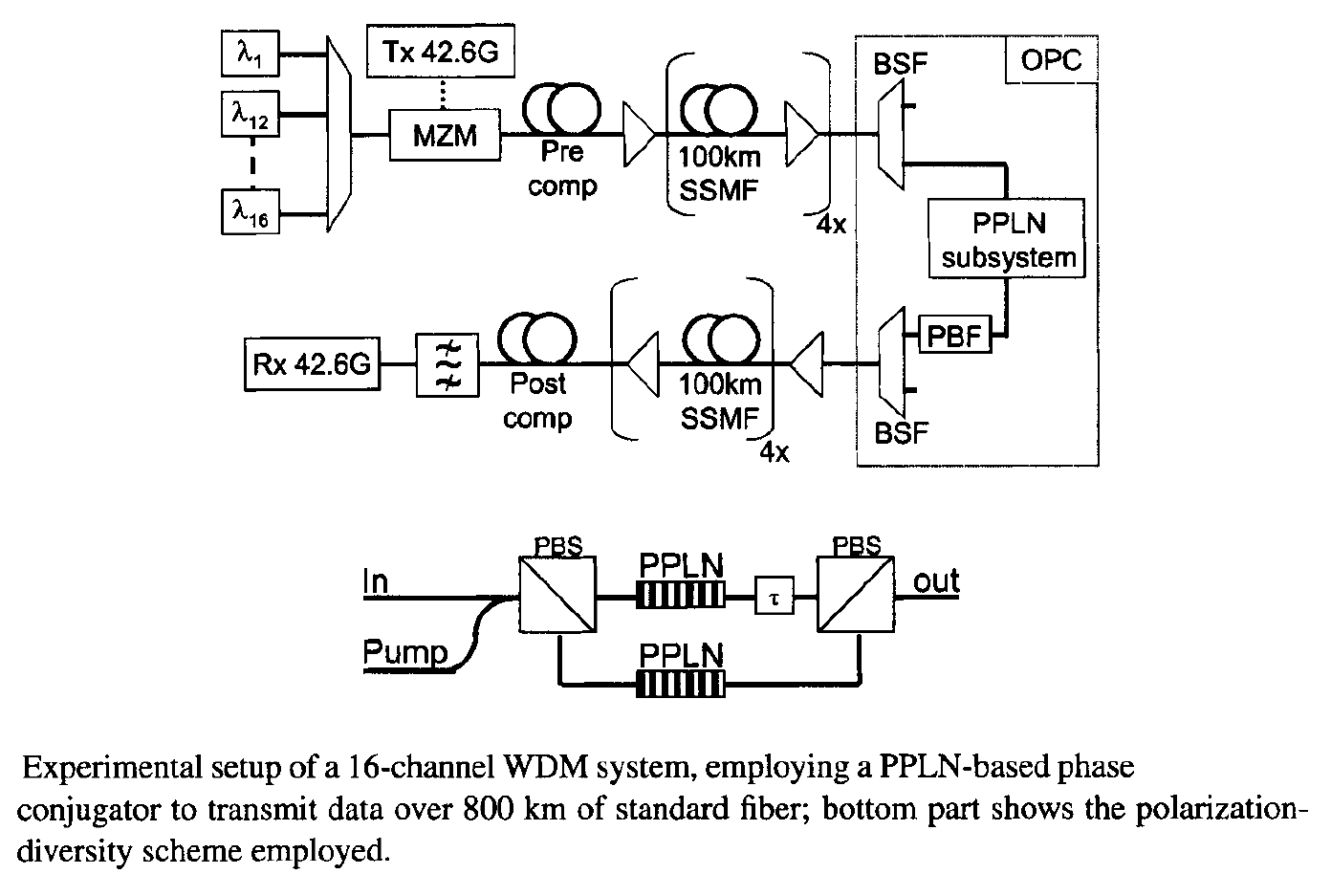For long-haul applications, one may ask whether the OPC technique can compensate the GVD acquired over thousands of kilometers. This question has been studied extensively through numerical simulations. In one set of simulations, a 10-Gb/s signal could be transmitted over 6,000 km when the average launch power was kept below 3 mW to reduce the effects of fiber nonlinearity. In another study, the amplifier spacing was found to play an important role; transmission over 9,000 km was feasible by keeping the amplifiers 40 km apart. The choice of the operating wavelength with respect to the zero-dispersion wavelength was also critical. In the case of anomalous dispersion (β2 < 0), periodic variations of the signal power along the fiber link can lead to the generation of additional sidebands through an instability known as sideband instability. This instability can be avoided if the dispersion parameter is relatively large [D > 16 ps/(km-nm)]. This is the case for standard fibers near 1.55 μm.

In general, the maximum transmission distance for the OPC technique depends on many factors such as FWM efficiency, input power, and amplifier spacing. If a suitably designed periodic dispersion map is used in combination with OPC, it is possible to suppress the sideband instability and increase the transmission distance to beyond 10,000 km. In a 2005 experiment, dispersion over 10,200 km was compensated for a 22 channels, operating at 20 Gb/s and separated by 50 GHz, using a mid-span PPLN-based phase conjugator. The 94.5-km recirculating loop employed standard fibers with a  dispersion of 16 ps/(km-nm), and its losses were compensated through Raman amplification. This experiment employed the RZ-DPSK format, and the OPC also helped in reducing the nonlinear phase noise.